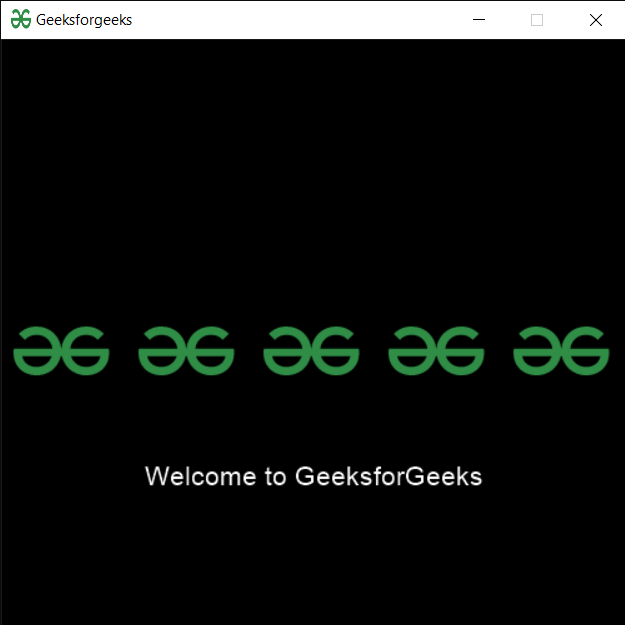# PYGLET – Drawing Multiple Sprites

• Last Updated : 26 May, 2022

In this article, we will see how we can draw multiple sprites on the window in PYGLET module in python. Pyglet is easy to use but powerful library for developing visually rich GUI applications like games, multimedia, etc. A window is a “heavyweight” object occupying operating system resources. Windows may appear as floating regions or can be set to fill an entire screen (fullscreen). A sprite is an instance of an image displayed on-screen. Multiple sprites can display the same image at different positions on the screen. Sprites can also be scaled larger or smaller, rotated at any angle and drawn at a fractional opacity. Image is loaded with the help of image module of pyglet.
Sprites can be batched together and drawn at once more quickly than if each of their draw methods were called individually. The following example creates one hundred ball sprites and adds each of them to a Batch. The entire batch of sprites is then drawn in one call

We can create a window, batch and sprite with the help of commands given below

```# creating a window
window = pyglet.window.Window(width, height, title)

# creating a batch
batch = pyglet.graphics.Batch()

# creating a sprite object
pyglet.sprite.Sprite(img, x, y)```

Below is the implementation

## Python3

 `# importing pyglet module``import` `pyglet``import` `pyglet.window.key as key``  ` `# width of window``width ``=` `500``  ` `# height of window``height ``=` `500``  ` `# caption i.e title of the window``title ``=` `"Geeksforgeeks"``  ` `# creating a window``window ``=` `pyglet.window.Window(width, height, title)``  ` `# text ``text ``=` `"Welcome to GeeksforGeeks"`` ` `# creating label with following properties``# font = cooper``# position = 250, 150``# anchor position = center``label ``=` `pyglet.text.Label(text,``                          ``font_name ``=``'Cooper'``,``                          ``font_size ``=` `16``,``                          ``x ``=` `250``, ``                          ``y ``=` `150``,``                          ``anchor_x ``=``'center'``, ``                          ``anchor_y ``=``'center'``)`  `# creating a batch``batch ``=` `pyglet.graphics.Batch()` `# loading geeksforgeeks image``image ``=` `pyglet.image.load(``'gfg.png'``)` `# creating a list of sprites object``sprites ``=` `[]` `# position of images``pos_x ``=` `10``pos_y ``=` `230` `for` `i ``in` `range``(``5``):``    ` `    ``# temporary sprite object``    ``temp ``=` `pyglet.sprite.Sprite(image, pos_x, pos_y, batch ``=` `batch)``    ` `    ``# append the sprite object to the list``    ``sprites.append(temp)``    ` `    ``# increment the x co-ordinate``    ``pos_x ``=` `pos_x ``+` `100`  `# on draw event``@window``.event``def` `on_draw():``      ` `    ``# clear the window``    ``window.clear()``      ` `    ``# draw the label``    ``label.draw()``    ` `    ``# draw the batch``    ``batch.draw()``      ` `# key press event    ``@window``.event``def` `on_key_press(symbol, modifier):``  ` `    ``# key "C" get press``    ``if` `symbol ``=``=` `key.C:``        ` `        ``# printing the message``        ``print``(``"Key : C is pressed"``)``        ` `# image for icon``img ``=` `image ``=` `pyglet.resource.image(``"gfg.png"``)` `    `  `# getting mouse button string``value ``=` `pyglet.window.mouse.buttons_string(``2` `| ``5``)`  `# setting image as icon``window.set_icon(img)``   ` `# start running the application``pyglet.app.run()`

Output :My Personal Notes arrow_drop_up